2023 NFL Defensive Rookie of the Year Odds
+350
3.5 to 1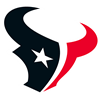Will Anderson
12.5% implied probability

+650
6.5 to 1Jalen Carter
7.5% implied probability

+900
9 to 1Devon Witherspoon
5.6% implied probability

+900
9 to 1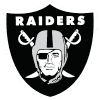Tyree Wilson
5.6% implied probability

+950
9.5 to 1Christian Gonzalez
5.4% implied probability

+1400
14 to 1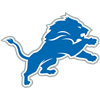Jack Campbell
3.7% implied probability

+1500
15 to 1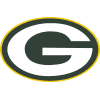Lukas Van Ness
3.5% implied probability

+1600
16 to 1Nolan Smith
3.3% implied probability

+1800
18 to 1Emmanuel Forbes
3.0% implied probability

+1800
18 to 1Will McDonald
3.0% implied probability

+2000
20 to 1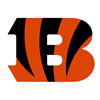Myles Murphy
2.7% implied probability

+2000
20 to 1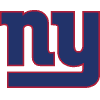Deonte Banks
2.7% implied probability

+2000
20 to 1Brian Branch
2.7% implied probability

+2000
20 to 1Felix Anudike-Uzomah
2.7% implied probability

+2500
25 to 1Calijah Kancey
2.2% implied probability

+2500
25 to 1Joey Porter
2.2% implied probability

+3000
30 to 1Trenton Simpson
1.8% implied probability

+3000
30 to 1Drew Sanders
1.8% implied probability

+3000
30 to 1BJ Ojulari
1.8% implied probability

+3500
35 to 1Bryan Bresee
1.6% implied probability

+3500
35 to 1Kelee Ringo
1.6% implied probability

+3500
35 to 1Keion White
1.6% implied probability

+3500
35 to 1Mazi Smith
1.6% implied probability

+3500
35 to 1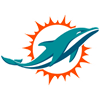Cam Smith
1.6% implied probability

+5000
50 to 1Derick Hall
1.1% implied probability

+5000
50 to 1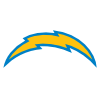Tuli Tuipulotu
1.1% implied probability

+5500
55 to 1Isaiah Foskey
1.0% implied probability

+6000
60 to 1Daiyan Henley
0.9% implied probability

+6000
60 to 1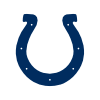Adetomiwa Adebawore
0.9% implied probability

+6000
60 to 1DJ Turner
0.9% implied probability

+6500
65 to 1Nick Herbig
0.9% implied probability

+6500
65 to 1Julius Brents
0.9% implied probability

+7000
70 to 1DeMarvion Overshown
0.8% implied probability

+7500
75 to 1Antonio Johnson
0.7% implied probability

+7500
75 to 1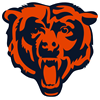Noah Sewell
0.7% implied probability

+10000
100 to 1Henry To'oTo'o
0.6% implied probability

+10000
100 to 1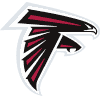Clark Phillips
0.6% implied probability

+10000
100 to 1Garrett Williams
0.6% implied probability

+10000
100 to 1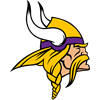Ivan Pace
0.6% implied probability

+10000
100 to 1Eli Ricks
0.6% implied probability

+10000
100 to 1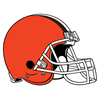Mohamoud Diabate
0.6% implied probability

+10000
100 to 1Tre'Vius Hodges-Tomlinson
0.6% implied probability

+10000
100 to 1Dee Winters
0.6% implied probability

+10000
100 to 1Tyrique Stevenson
0.6% implied probability

+10000
100 to 1Isaiah Moore
0.6% implied probability

+10000
100 to 1Micah Baskerville
0.6% implied probability

+10000
100 to 1Jaylon Jones
0.6% implied probability

+10000
100 to 1Kyu Blu Kelly
0.6% implied probability

+10000
100 to 1Cam Jones
0.6% implied probability

+10000
100 to 1Dorian Williams
0.6% implied probability

+15000
150 to 1Alex Austin
0.4% implied probability